PHY_10_62_V2_DM_Faraday law of induction
Оценка 4.6

# PHY_10_62_V2_DM_Faraday law of induction

Оценка 4.6
doc
08.05.2020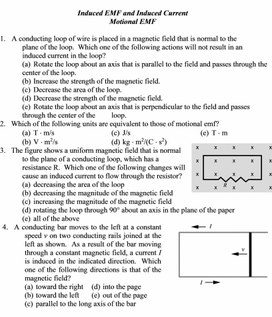PHY_10_62_V2_DM_Faraday law of induction.doc

Induced EMF and Induced Current

Motional EMF

1.   A conducting loop of wire is placed in a magnetic field that is normal to the plane of the loop.  Which one of the following actions will not result in an induced current in the loop?

(a)  Rotate the loop about an axis that is parallel to the field and passes through the center of the loop.

(b)  Increase the strength of the magnetic field.

(c)  Decrease the area of the loop.

(d) Decrease the strength of the magnetic field.

(e)  Rotate the loop about an axis that is perpendicular to the field and passes through the center of the       loop.

2.   Which of the following units are equivalent to those of motional emf?

(a)  T Ч m/s                               (c)  J/s                                      (e)  T Ч m

(b)  V Ч m2/s                             (d) kg Ч m2/(C Ч s2)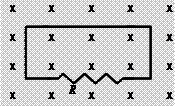3.   The figure shows a uniform magnetic field that is normal to the plane of a conducting loop, which has a resistance R.  Which one of the following changes will cause an induced current to flow through the resistor?

(a)  decreasing the area of the loop

(b)  decreasing the magnitude of the magnetic field

(c)  increasing the magnitude of the magnetic field

(d) rotating the loop through 90° about an axis in the plane of the paper

(e)  all of the above

 4.   A conducting bar moves to the left at a constant speed v on two conducting rails joined at the left as shown.  As a result of the bar moving through a constant magnetic field, a current I is induced in the indicated direction.  Which one of the following directions is that of the magnetic field?       (a)  toward the right     (d) into the page       (b)  toward the left       (e)  out of the page       (c)  parallel to the long axis of the bar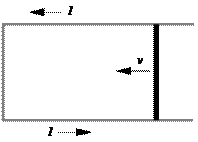5.   A 2.0-kg rod that has a length of 1.0 m and a resistance of 4.0 slides with constant speed down a pair of frictionless vertical conducting rails that are joined at the bottom.  Other than the rod, the rest of the circuit is resistanceless.  A uniform magnetic field of magnitude 3.0 T is perpendicular to the plane formed by the rod and the rails as shown.  Determine the speed of the rod.       (a)  0.38 m/s                             (d) 5.6 m/s       (b)  0.90 m/s                             (e)  8.7 m/s       (c)  2.6 m/s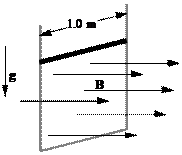6.   A 0.45-m metal rod moves 0.11 m in a direction that is perpendicular to a 0.80-T magnetic field in an elapsed time of 0.036 s.  Assuming that the acceleration of the rod is zero m/s2, determine the emf that exists between the ends of the rod.

(a)  1.1 V                                 (d) 9.1 ґ 10–5 V

(b)  0.27 V                               (e)  This cannot be determined without knowing the

(c)  0.076 V                                   orientation of the rod relative to the magnetic field.

Magnetic Flux

7.   A 0.50-T magnetic field is directed perpendicular to the plane of a circular loop of radius 0.25 m. What is the magnitude of the magnetic flux through the loop?

(a)  0.049 Wb                          (c)  0.20 Wb                            (e)  zero Wb

(b)  0.098 Wb                          (d) 0.39 Wb

8.   The Earth’s magnetic field passes through a square tabletop with a magnitude of 4.95 ґ 10-5 T and is directed at an angle of 165° relative to the normal of the tabletop.  If the tabletop has 1.50-m sides, what is the magnitude of the magnetic flux through it?

(a)  1.08 ґ 10–4 Wb                 (c)  2.88 ґ 10–5 Wb                       (e)  3.30 ґ 10–6 Wb

(b)  7.11 ґ 10–5 Wb                 (d) 1.92 ґ 10–5 Wb

9.   A circular copper loop is placed perpendicular to a uniform magnetic field of 0.50 T.  Due to external forces, the area of the loop decreases at a rate of 1.26  10–3 m2/s.  Determine the induced emf in the loop.

(a)  3.1 ґ 10–4 V                      (c)  1.2 ґ 10–3 V                            (e)  3.1 V

(b)  6.3 ґ 10–4 V                      (d) 7.9 ґ 10–3 V

10. A conducting loop has an area of 0.065 m2 and is positioned such that a uniform magnetic field is perpendicular to the plane of the loop.  When the magnitude of the magnetic field decreases to 0.30 T in 0.087 s, the average induced emf in the loop is 1.2 V.  What is the initial value of the magnetic field?

(a)  0.42 T                                (c)  0.87 T                                (e)  1.9 T

(b)  0.75 T                                (d) 1.2 T

11. A uniform magnetic field passes through two areas, A1 and A2.  The angles between the magnetic field and the normals of areas A1 and Aare 30.0° and 60.0°, respectively.  If the magnetic flux through the two areas is the same, what is the ratio A1/A2?

(a)  0.577                                 (c)  1.00                                   (e)  1.73

(b)  0.816                                 (d) 1.23

12. A 150-turn solenoid carries a current of 12 A.  The radius of the solenoid is 0.050 m; and its length is 0.18 m.  Determine the magnetic flux through the circular cross-sectional area at the center of the solenoid.

(a)  1.8 ґ 10–5 Wb                   (c)  4.3 ґ 10–4 Wb                   (e)  2.2 ґ 10–3 Wb

(b)  9.9 ґ 10–5 Wb                   (d) 7.0 ґ 10–4 Wb

Faraday’s Law of Electromagnetic Induction

13. A magnetic field is directed perpendicular to the plane of a 0.15-m ґ 0.30-m rectangular coil consisting of 120 loops of wire.  To induce an average emf of -1.2 V in the coil, the magnetic field is increased  from 0.1 T to 1.5 T during a time interval t.  Determine t.

(a)  0.053 s                               (c)  1.6 s                                   (e)  7.6 s

(b)  0.13 s                                 (d) 6.3 s14.             The area of a 333-turn conducting coil is 7.85 ґ 10–3 m2.  The resistance of the coil is 10.4 .  If the coil is oriented as shown in a magnetic field B, at what rate in T/s should the magnitude of B change to induce a current of 2.50 ґ 10–3 A in the coil?

(a)  0.0155 T/s                         (c)  0.228 T/s                           (e)  1.52 T/s

(b)  0.0996 T/s                         (d) 0.757 T/s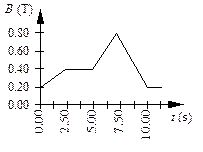15. A circular coil of wire has 25 turns and has a radius of 0.075 m.  The coil is located in a variable magnetic field whose behavior is shown on the graph.  At all times, the magnetic field is directed at an angle of 75° relative to the normal to the plane of a loop.  What is the average emf induced in the coil in the time interval from t = 5.00 s to 7.50 s?

(a)  -18 mV                             (d) -140 mV

(b)  -49 mV                             (e)  -180 mV

(c)  -92 mV

Questions 16 through 19 pertain to the situation described below:

 The figure shows a uniform, 3.0-T magnetic field that is normal to the plane of a conducting, circular loop with a resistance of 1.5  and a radius of 0.024 m.  The magnetic field is directed out of the paper as shown.  Note:  The area of the non-circular portion of the wire is considered negligible compared to that of the circular loop.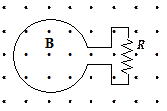16. What is the magnitude of the average induced emf in the loop if the magnitude of the magnetic field is doubled in 0.4 s?

(a)  0.43 V                               (c)  0.014 V                             (e)  0.038 V

(b)  0.65 V                               (d) 0.027 V

17. What is the average current around the loop if the magnitude of the magnetic field is doubled in 0.4 s?

(a)  2.8 * 10–3 A, clockwise                             (d) 9.0 * 10–3 A, clockwise

(b)  4.5 * 10–3 A, clockwise                             (e)  9.0 * 10–3 A, counterclockwise

(c)  4.5 * 10–3 A, counterclockwise

18. If the magnetic field is held constant at 3.0 T and the loop is pulled out of the region that contains the field in 0.2 s, what is the magnitude of the average induced emf in the loop?

(a)  8.6 * 10–3 V                      (c)  2.7 * 10–2 V                       (e)  6.4 * 10–2 V

(b)  9.8 * 10–2 V                      (d) 5.4 * 10–2 V

19. If the magnetic field is held constant at 3.0 T and the loop is pulled out of the region that contains the field in 0.2 s, at what rate is energy dissipated in R?

(a)  1.8 * 10–2 W                      (c)  3.8 * 10–3 W                            (e)  4.9 * 10–4 W

(b)  3.6 * 10–2 W                      (d) 2.7 * 10–4 W

## If the magnetic flux through the two areas is the same, what is the ratio## What is the magnitude of the average induced emf in the loop if the magnitude of the magnetic field is doubled in 0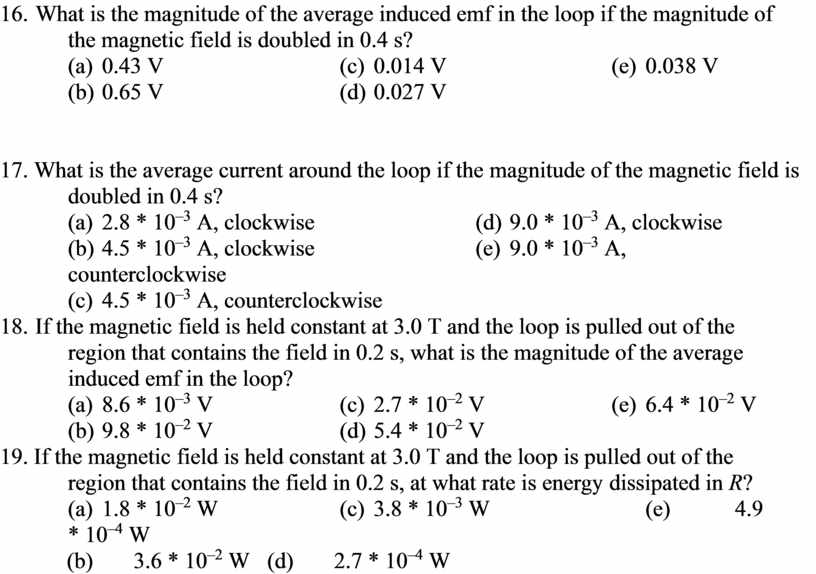Скачать файл